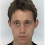## Wednesday, January 16, 2013

### A sampling gem: sampling from $\ell_p$ balls.

A while back, I had written about uniform sampling from the $d$-simplex. In brief, sample exponentially distributed random variables, and normalize them. Note that the simplex is one piece of the $d$-dimensional $\ell_1$ unit sphere.

I had also mentioned a similar trick to sample from the ($\ell_2$) unit sphere: sample Gaussian random variables, and normalize them.

As I discovered today, these are really just special cases of a much more general result that includes both of these approaches, as well as providing a general way to sample from the $d$-dimensional unit ball (as well as sphere) under any $\ell_p$ norm.

The result is due to Barthe, Guedon, Mendelson and Naor, and is breathtakingly elegant. Here it is:

To sample from the unit ball under the $\ell_p$ norm in $d$ dimensions, randomly sample $d$ coordinates $x_1, \ldots, x_d$ i.i.d from a density proportional to $\exp(-|x|^p)$. Also sample $Y$ from the exponential distribution with parameter $\lambda = 1$. Then the desired sample is
$$\frac{(x_1, x_2, \ldots, x_d)}{\Bigl(\sum_i |x_i|^p + Y\Bigr)^{1/p}}$$
Notice that merely eliminating the $Y$ recovers the procedure for sampling from the unit sphere and includes both of the above sampling procedures as a special case. It's far more efficient than either rejection sampling, or even the metropolis-sampling method from the theory of sampling from convex bodies. Also, if you only want to sample from an $\ell_2$ ball you can do it without this hammer using the sphere sampling technique and a nonuniform sampling of the length, but this seems so much more elegant.

1.Can you elaborate more on Y ?

2.It's basically what I said in the post. Choose Y distributed as exp(-x)

3.Hi,
Is there any intuitive explanation about the use of the exponential(1) distribution to "choose" the samples' distance from the origin?

4.It's a good question. I'm not sure. the paper is a little cryptic.

5.(and also, shouldn't it be a p-th root, instead of a square one?)

6.Ah you're right. sorry for the mistake.

7.Quick note: for densities of the form exp(-|x|^p), the normalizing constant is 2/p * gamma(1/p). It comes in handy for some sampling algorithms and for the use of such densities in inference (e.g. Lindsey's method, as in http://yaroslavvb.com/papers/efron-semiparametric.pdf).